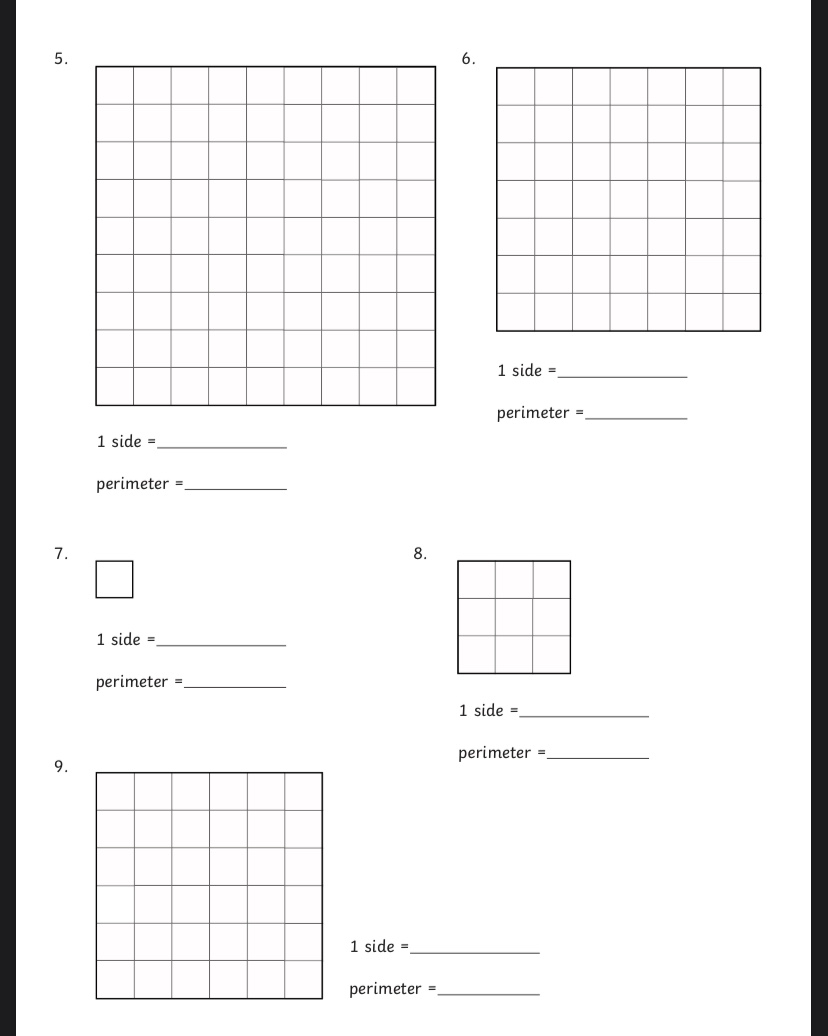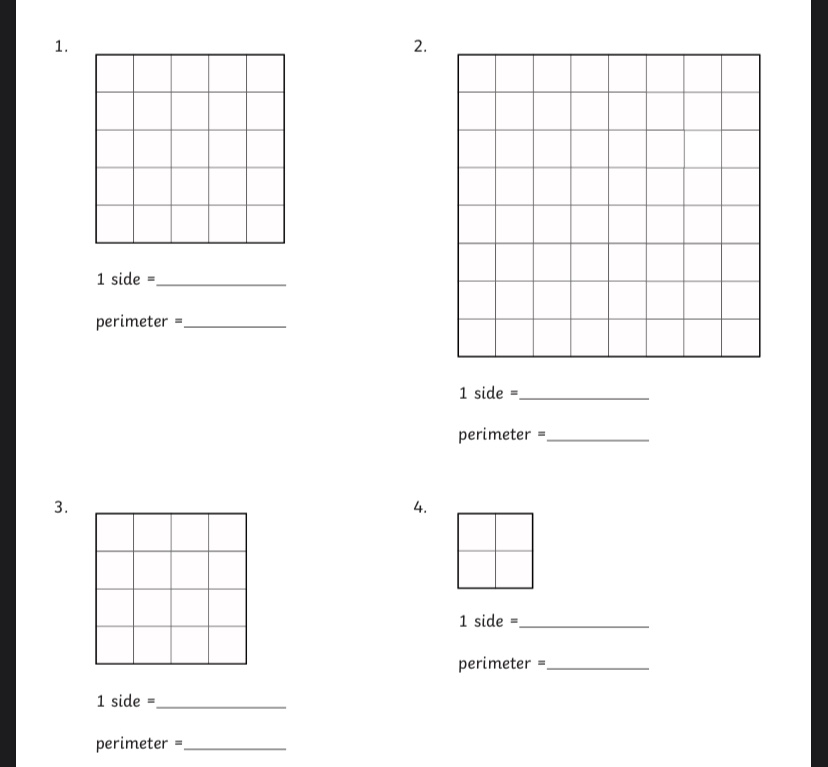# Y4 Maths- Wednesday 22nd April

Good morning everyone! Hope you are all OK?

Well done for yesterdays maths work, I loved seeing all of your pictures!

Sorry for the late post, my internet hasn’t been working very well.

Today we are going to be looking at perimeter of squares and rectangles.

Perimeter means the length of the outside of the shape. Imagine a fence that goes around the outside of your garden, the total length of the fence is the perimeter.

We are looking at squares and rectangles today. We know that a square has 4 sides which are all the same length and a rectangle has 4 sides where 2 sides that are opposite or parallel to each other are the same. We can use this information to help us calculate the perimeter.

Use the squares to help you calculate the length of each side (remember 1 square = 1cm) or if you have printed the sheet and have a ruler you can measure it.

Add the 4 side lengths together to calculate the perimeter.

For example:

A square has 4 sides which are 5cm long

we need to add the 4 sides up :

5cm + 5cm +5cm +5cm = 20cm  or 5cm x 4 = 20cm

A rectangle has 2 sides which equal 3cm and 2 sides which equal 6cm

We need to add the lengths of all 4 sides together:

3cm + 3cm +6cm +6cm = 18cm

The perimeter is 18cm.perimeter

### 19 thoughts on “Y4 Maths- Wednesday 22nd April”

•22nd April 2020 at 10:17 am

This morning Rihanna did ultimate times table missing number challenge her score was 143 out of 144 well done Rihanna msg for Mrs denny

•22nd April 2020 at 12:05 pm

1.
1 side
5 perimeter
2.
1 side
8 perimeters
3.
1 side
4 perimeters
4.
1 sides
2 perimeters
5.
1 side
9 perimeters
6.
1 side
7 perimeters
7.
1 side
1 perimeter
8.
1 side
3 perimeters
9.
1 side
6 perimeters

•22nd April 2020 at 9:50 pm

Fantastic understanding Brooke. Make sure you are enjoying the sunshine and having fun.

•22nd April 2020 at 12:18 pm

1, 1 side = 5cm perimeter = 20cm
2, 1 side = 8cm perimeter = 32cm
3, 1 side = 4cm perimeter = 16cm
4, 1 side=2cm perimeter = 8cm
5, 1 side = 9cm perimeter = 36cm
6, 1 side = 7cm perimeter = 28cm
7, 1 side = 1cm perimeter = 4cm
8, 1 side = 3cm perimeter =12cm
9, 1 side = 6cm perimeter = 24cm

Rectangular
1, Side 1 =1cm Side 2 = 6cm perimeter =14cm
2, Side 1 = 3cm Side 2 = 7cm perimeter =20cm
3, Side 1 = 5cm Side 2 = 4cm perimeter = 18cm
4, Side 1= 3cm Side 2 = 10cm perimeter =26cm
5,Side 1= 5cm Side 2 =9cm Perimeter = 28cm
6, Side 1 = 2cm Side 2 = 6cm Perimeter = 16cm
7 Side 1 = 4cm Side 2 = 9cm perimeter = 26cm
8, Side 1 = 2cm Side 2 = 7cm perimeter = 18cm
9 Side 1 = 6cm Side 2 = 9cm perimeter = 30cm
10 Side 1 =5cm Side 2 = 7cm perimeter = 24cm
11 Side 1 3cm Side 2 = 8cm perimeter = 22cm
12 Side 1 = 5cm Side 2 = 7cm perimeter = 24cm

•22nd April 2020 at 3:40 pm

Brilliant Ben, well done. Lovely clear working out.

•22nd April 2020 at 12:19 pm

1) 5 cm per side. Perimeter of 20 cm. Area of 25 cm2.
2) 8 cm per side. Perimeter of 32 cm. Area of 64 cm2.
3) 4 cm per side. Perimeter of 16 cm. Area of 16 cm2.
4) 2 cm per side. Perimeter of 8 cm. Area of 4 cm2.
5) 9 cm per side. Perimeter of 36 cm. Area of 81 cm2.
6) 7 cm per side. Perimeter of 28 cm. Area of 49 cm2.
7) 1 cm per side. Perimeter of 4 cm. Area of 1 cm2.
8) 3 cm per side. Perimeter of 12 cm. Area of 9 cm2.
9) 6 cm per side. Perimeter of 24 cm. Area of 36 cm2

•22nd April 2020 at 3:39 pm

Fantastic work, Franek. I love that you have calculated the area too.

•22nd April 2020 at 3:10 pm

Perimeter of squares.
1. side = 5cm perimeter = 20cm
2. side = 8cm Perimeter = 32cm
3. side = 4cm Perimeter = 20cm
4. side = 2cm Perimeter = 8cm
5. side = 9cm Perimeter = 36cm
6. side = 7cm Perimeter = 21cm
7. side = 1cm Perimeter = 4cm
8. side = 3cm Perimeter = 12cm
9. side = 6cm Perimeter = 24cm

perimeter of rectangles.

1. side 1 = 1cm side 2 = 6cm Perimeter = 14cm
2. side 1 = 3cm side 2 = 7cm Perimeter = 20cm
3. side 1 = 5cm side 2 = 4cm Perimeter = 18cm
4. side 1 = 3cm side 2 = 10cm Perimeter = 26cm
5. side 1 = 5cm side 2 = 9cm Perimeter = 28cm
6. side 1 = 2cm side 2 = 6cm Perimeter = 16cm
7. side 1 = 4cm side 2 = 9cm Perimeter = 26cm
8. side 1 = 2cm side 2 = 7cm Perimeter = 18cm
9. side 1 = 6cm side 2 = 9cm Perimeter = 30cm
10. side 1 = 5cm side 2 = 7cm Perimeter = 24cm
11. side 1 = 3cm side 2 = 8cm Perimeter = 22cm
12. side 1 = 5cm side 2 = 7cm Perimeter = 24cm

•22nd April 2020 at 3:36 pm

Lovely clear working out Hollie, well done. I can see you understand how to solve perimeter.

•22nd April 2020 at 7:10 pm

(1)5cm is 1 side.perimeter 20cm
(2)8cm is 1 side.perimeter 32cm
(3)4cm is 1 side.perimeter 20cm
(4)2cm is 1 side.perimeter 8cm
(5)9cm is 1 side.perimeter 36cm
(6)7cm is 1 side.perimeter 21cm
(7)1cm is 1 side.perimeter 4cm
(8)3cm is 1 side.perimeter 12cm
(9)6cm is 1 side.perimeter 24cm

•22nd April 2020 at 9:46 pm

Excellent understanding Michael good to see you back on here

•22nd April 2020 at 3:16 pm

Shapes perimeters.
1. Sides-5cm
Perimeter-20cm

2. Sides-8cm
Perimeter-32cm

3. Sides-4cm
Perimeter- 16cm

4. Sides-2cm
Perimeter-8cm

5. Sides-9cm
Perimeter-36cm.

6. Sides-7cm
Perimeter-28cm

7.Sides-1cm Perimeter-4cm

8. Sides-3cm
Perimeter-12cm
9. Sides-6cm
Perimeter-24cm

Rectangular
1. Side 1- 6cm
2- 1cm
Perimeter -14cm

2. Side 1-7cm
2- 3cm
Perimeter-20cm

3. Side 1-4cm
2- 5cm
Perimeter-18cm#

6. Side 1-10cm
2- 3cm
Perimeter-26cm

7. Side 1-4cm
2-9cm
Perimeter-26cm

8. Side 1-7cm
2-2cm
Perimeter-18cm

9. Side 1-9cm
2-6cm
Perimeter-30cm

10.Side 1- 7cm
2- 5cm
Perimeter-24cm

11.Side 1- 8cm
2-3cm
Perimeter-24cm

12. Size 1- 5cm
2-7cm
Perimeter-24cm

•22nd April 2020 at 3:35 pm

Excellent work Hargun! I can see that you clearly understand how to calculate perimeter.

•23rd April 2020 at 11:37 am

1. side=5cm permiter=20cm
2. side=8cm permiter=32cm
3. side=4cm permiter=16cm
4. side=2cm permiter=8cm
5. side=9cm permiter=36cm
6. side=7cm permiter=28cm
7. side=1cm permiter=4cm
8. side=3cm permiter=12cm
9. side=6cm permiter=24cm

•23rd April 2020 at 1:21 pm

Great understanding of Perimeter Damian

•23rd April 2020 at 1:46 pm

The Perimeter of Rectangles
1. Side 1 = 1cm, side 2 = 6cm, perimeter = 14cm
2. Side 1 = 3cm, side 2 = 7cm, perimeter = 20cm
3. Side 1 = 5cm, side 2 = 4cm, perimeter = 18cm
4. Side 1 = 3cm, side 2 = 10cm, perimeter = 26cm
5. Side 1 = 5cm, side 2 = 9cm, perimeter = 28cm
6. Side 1 = 2cm, side 2 = 6cm, perimeter = 16cm
7. Side 1 = 4cm, side 2 = 9cm, perimeter = 26cm
8. Side 1 = 2cm, side 2 = 7cm, perimeter = 18cm
9. Side 1 = 6cm, side 2 = 9cm, perimeter = 30cm
10. Side 1 = 5cm, side 2 = 7cm, perimeter = 24cm
11. Side 1 = 3cm, side 2 = 8cm, perimeter = 22cm
12. Side 1 = 5cm, side 2 = 7cm, perimeter = 24cm

•23rd April 2020 at 3:25 pm

1. 1 side=5cm perimeter=20cm
2. 1 side=8cm perimeter=32cm
3. 1 side=4cm perimeter=16cm
4. 1 side=2cm perimeter=8cm
5. 1 side=9cm perimeter=36cm
6. 1 side=7cm perimeter=28cm
7. 1 side=1cm perimeter=4cm
8. 1 side=3cm perimeter=12
9. 1 side=6cm perimeter=24cm

•23rd April 2020 at 3:28 pm

The Perimeter of Squares sheet 1

1 side=5cm perimeter=20cm
1 side=8cm perimeter=32cm
1 side=4 cm perimeter=16cm
1 side=2cm perimeter= 8cm
1 side=9cm perimeter=36cm
1 side=7cm perimeter=28cm
1 side=1cm perimeter=4cm
1 side=3cm perimeter=12cm
1side=6cm perimeter=24cm

The perimeter of squares Sheet 2

1 side=9 cm perimeter=36cm
1 side=1cm perimeter=4cm
1 side=3cm perimeter=12cm
1 side=6cm perimeter=24cm
1 side=10cm perimeter=40cm
1 side=2cm perimeter=8cm
1 side=5cm perimeter=20cm
1 side=8cm perimeter=32cm
1side=1.5cm perimeter=6cm
1 side=9.5cm perimeter=38cm
1 side=6.5cm perimeter=26cm
1 side=7.5cm perimeter=30cm
1 side=2.5cm perimeter=10cm
1 side=10.5cm perimeter=42cm
1 side=3.5cm perimeter=14cm
1 side=8.5cm perimeter=34cm

•